Скачать презентацию 15 March 2018 Gravitational Potential Energy Objectives Be

319d145d361006a5d54ddfc9d9e01d8b.ppt

• Количество слайдов: 7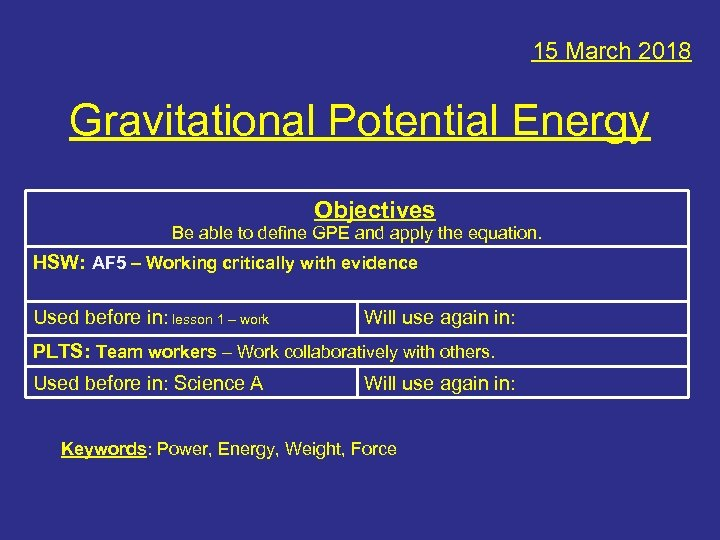15 March 2018 Gravitational Potential Energy Objectives Be able to define GPE and apply the equation. HSW: AF 5 – Working critically with evidence Used before in: lesson 1 – work Will use again in: PLTS: Team workers – Work collaboratively with others. Used before in: Science A Will use again in: Keywords: Power, Energy, Weight, Force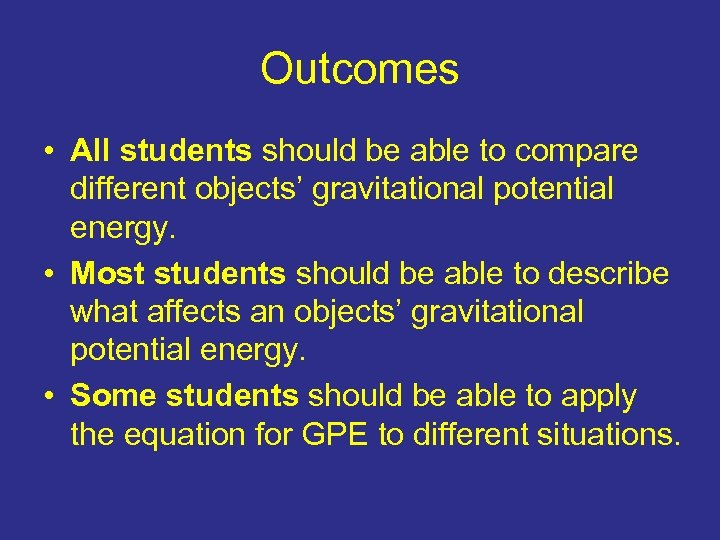Outcomes • All students should be able to compare different objects’ gravitational potential energy. • Most students should be able to describe what affects an objects’ gravitational potential energy. • Some students should be able to apply the equation for GPE to different situations.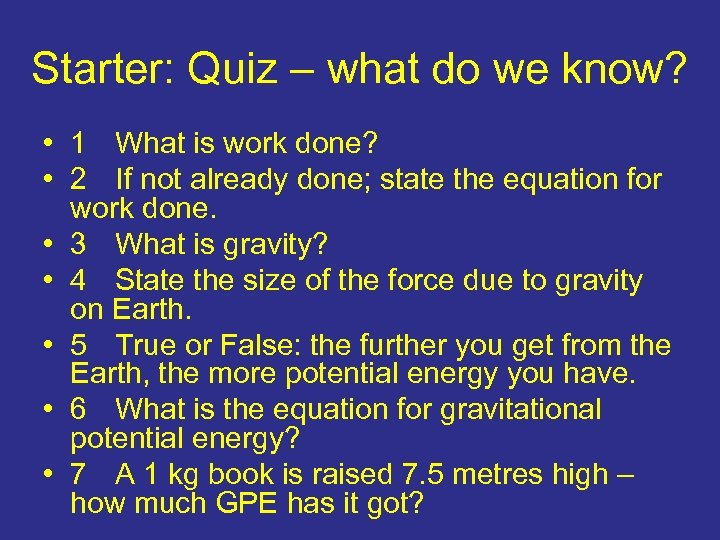Starter: Quiz – what do we know? • 1 What is work done? • 2 If not already done; state the equation for work done. • 3 What is gravity? • 4 State the size of the force due to gravity on Earth. • 5 True or False: the further you get from the Earth, the more potential energy you have. • 6 What is the equation for gravitational potential energy? • 7 A 1 kg book is raised 7. 5 metres high – how much GPE has it got?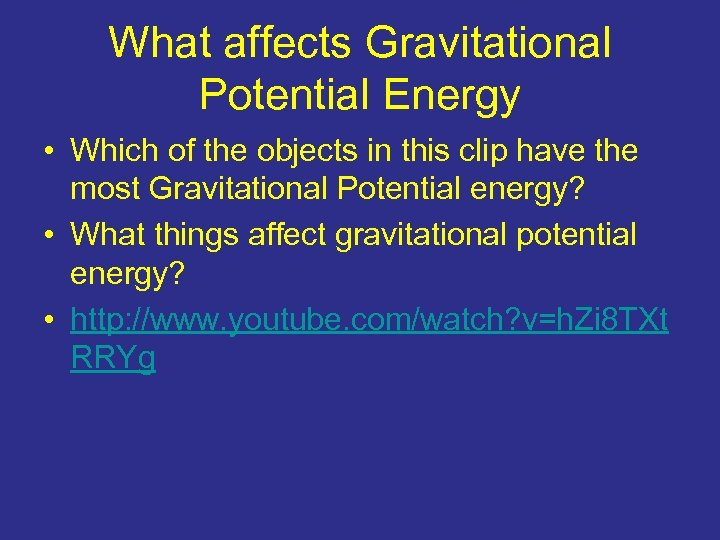What affects Gravitational Potential Energy • Which of the objects in this clip have the most Gravitational Potential energy? • What things affect gravitational potential energy? • http: //www. youtube. com/watch? v=h. Zi 8 TXt RRYg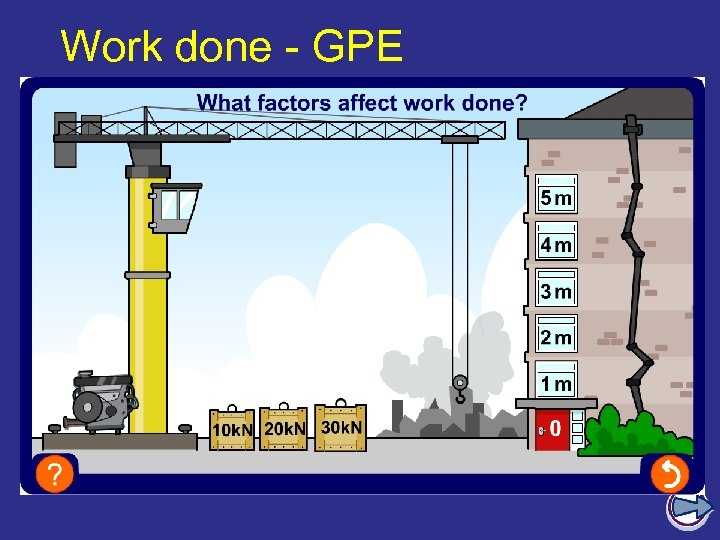Work done - GPE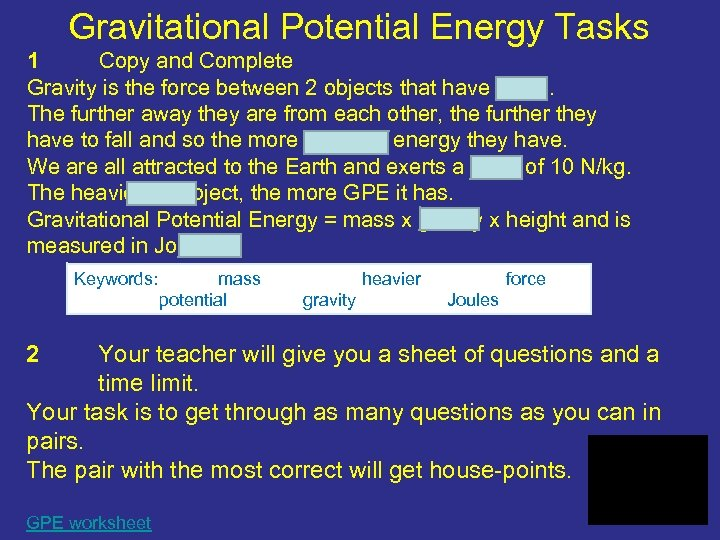Gravitational Potential Energy Tasks 1 Copy and Complete Gravity is the force between 2 objects that have mass. The further away they are from each other, the further they have to fall and so the more potential energy they have. We are all attracted to the Earth and exerts a force of 10 N/kg. The heavier an object, the more GPE it has. Gravitational Potential Energy = mass x gravity x height and is measured in Joules. Keywords: 2 mass potential heavier gravity force Joules Your teacher will give you a sheet of questions and a time limit. Your task is to get through as many questions as you can in pairs. The pair with the most correct will get house-points. GPE worksheet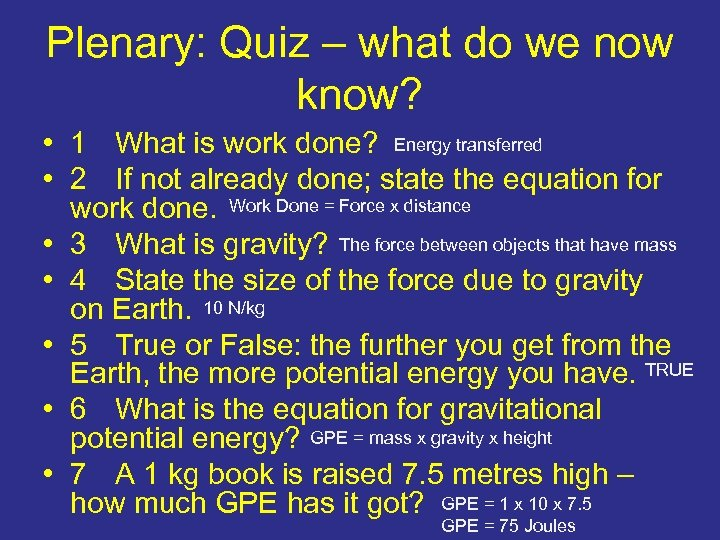Plenary: Quiz – what do we now know? • 1 What is work done? Energy transferred • 2 If not already done; state the equation for work done. Work Done = Force x distance • 3 What is gravity? The force between objects that have mass • 4 State the size of the force due to gravity on Earth. 10 N/kg • 5 True or False: the further you get from the Earth, the more potential energy you have. TRUE • 6 What is the equation for gravitational potential energy? GPE = mass x gravity x height • 7 A 1 kg book is raised 7. 5 metres high – how much GPE has it got? GPE = 1 x 10 x 7. 5 GPE = 75 Joules